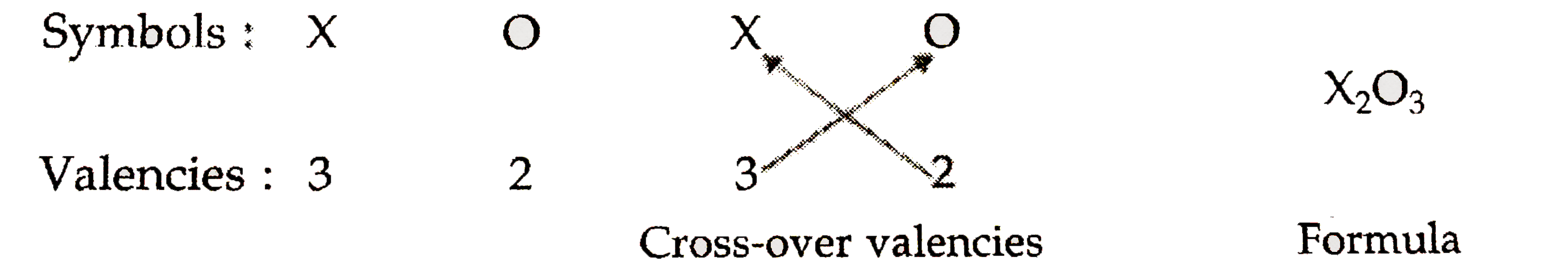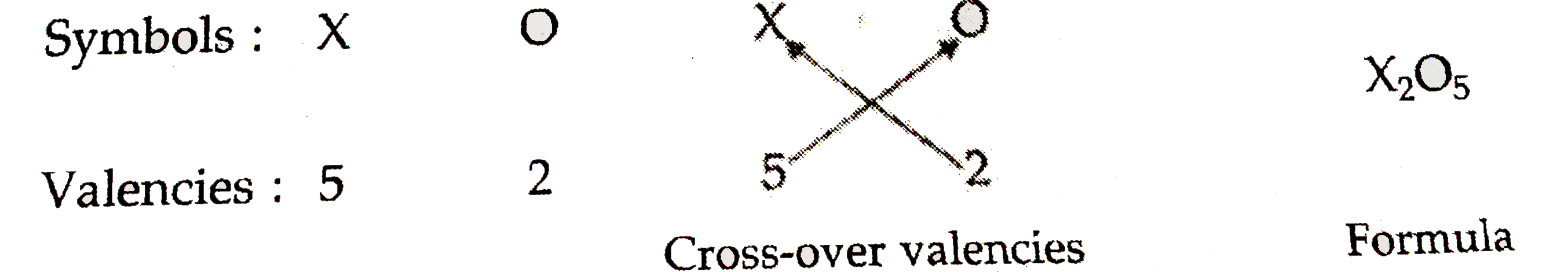# An element X shows two valencies of 3 and 5. Work out the formulae of two oxides of this element.

1.4k views

closed
An element X shows two valencies of 3 and 5. Work out the formulae of two oxides of this element.

by (40.8k points)
selected by

In order to find out the formulae of the oxides of element X , we should know the valency of oxygen element (because an element combines with oxygen element to form the oxide). Knowing that the valenecy of oxygen is 2, we can write down the formulate of the two oxides of element X as follows :
(a) Formula of oxide of element X when its valenecy is 3.Thus, the formula of oxide of elemene X when its valency is 3 is X_(2)O_(3).
(b) Formula of oxide of element X When its valency is 5Thus, the formula of oxide of element X when its valency is 5 is X_(2)O_(5).